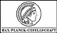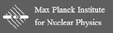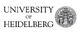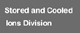Superordinated navigation: MPIK Homepage  |  Home  |  Deutsch  |  Sitemap  |  Search  |  Contact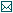Contact

Tel.: +49 6221 516-851
Fax: +49 6221 516-852
Max Planck Institute for Nuclear Physics
P.O. Box 10 39 80
69029 Heidelberg
Max Planck Institute for Nuclear Physics
Saupfercheckweg 1
Building: Gentner lab,
room 134
69117 Heidelberg

# THe-TRAP Project

## Physical basics

While a mass measurement in everyday life is often based on measuring a force, for example personal or postal scales, this concept is not sufficient for our required accuracy and is no longer applicable to atomic particles. To get an idea of the desired accuracy, two comparisons with everyday life can be made. E.g. the mass of the currently largest commercial aircraft the Airbus A380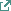with a maximum takeoff weight of 560 tons. With an accuracy of 10-11 it can be measured with an uncertainty of 5.6 mg. This is approximately the mass of one human hair. Applied to the Earth circumference of 40.000 km, this would have to be measured with a precision of 0.4mm.Fig. 3.1: The graph shows an ideal hyperbolic Penning trap. It consists of three hyperbolic electrodes (endcap, ring, endcap), which generate an ideal quadrupole potential. The electric field generated by the electrodes is overlaid with a uniform magnetic field B0 in the z direction.

Instead of a force measurement, Penning trap mass spectrometry measures massdependent frequencies. In a uniform magnetic field the Lorentz force confines charged particle on a circular orbit. The frequency of the movement

νc = 1/2π ⋅ q/m ⋅ B0(3.1)

is called free-space cyclotron frequency νc. Here q is the known charge, m the derived mass of the particle and B0 the magnetic field. For a full spatial storage of the ion perpendicular to the plane of the cyclotron motion an electric field must be applied, which (see Figure 3.1) is generated by hyperbolic electrodes. This changes the motion of the ion in the trap and the three resulting eigenmotions can be described by three independent frequencies. These are

• the axial frequency

νz = 1/2π ⋅ √(q/m ⋅ U0/d2)(3.2)

• the reduced cyclotron frequency

ν+ = νc/2 + √(ν2c/4 - ν2z/2)(3.3)

• and the magnetron frequency

ν- = νc/2 - √(ν2c/4 - ν2z/2)(3.4)

Here U0 is the voltage applied to the electrode and d is a characteristic trap size, which for hyperbolic traps results from the boundary conditions imposed by the electrodes. The invariance theorem states that the free-space cyclotron frequency can be calculated by summing up of the independent frequencies in quadrature:

ν2c = ν2z + ν2+ + ν2-(3.5)

In the experiment, νc will be determined for tritium and helium-3 and from this we get the frequency ratio for these elements, which leads to a mass ratio, if the magnetic field is stable enough.